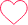# Find the Greatest of the Three Numbers in C

## Find the Greatest of Three Numbers in C

Given three integers num1, num2 and num3 as inputs. The objective is to write a code to Find the Greatest of the Three Numbers in C Language. To do so we’ll check the numbers with each other and print the largest of them all.

```Example
Input : num1 = 2 num2 = 9 num3 = 4
Output : 9
```## Find the Greatest of the Three Numbers in C

Given Three integer inputs num1, num2 and num3, the objective is ti write a code to Find the Largest of the Three Numbers in C Language. In this article we will see a  C program to Find Greatest of three numbers in C. We will use if else conditions and ternary operator too to find the same. Here are some of the methods to solve the above mentioned problem,

• Method 1: Using if-else Statements 2
• Method 2: Using if-else Statements 2
• Method 3: Using Ternary Operator

We’ll discuss the above mentioned methods in the sections below in depth.

### C Code

Run
```#include <stdio.h>
int main ()
{
int num1, num2, num3;

num1=12,num2=17,num3=19;

//checking if num1 is greatest
if (num1 >= num2 && num1 >= num3)
printf("%d is the greatest", num1);

//checking if num2 is greatest
else if(num2 >= num1 && num2 >= num3)
printf("%d is the greatest", num2);

//checking if num2 is greatest
else if(num3 >= num1 && num3 >= num2)
printf("%d is the greatest", num3);

return 0;
}
```

### Output

`19 is the greatest`

### C Code

Run
```#include <stdio.h>
int main ()
{
int num1, num2, num3;

num1=13,num2=45,num3=27;

//comparing num1 with other numbers
if ((num1 >= num2) && (num1 >= num3))
printf("%d is the greatest", num1);

//comparing num2 with other numbers
else if ((num2 >= num1) && (num2 >= num3))
printf("%d is the greatest", num2);

// num3 has to be greatest then if not above
else
printf("%d is the greatest", num3);

return 0;
}
```

### Output

`45 is the greatest`

### Method 3: Using Ternary Operator

In this method we use the knowledge of Ternary Operators in C

### C Code

Run
```#include <stdio.h>
int main ()
{
int num1, num2, num3;

num1=12,num2=98,num3=84;

int temp, result;

// find the largest b/w num1 and num2 & store in temp
temp = num1>num2 ? num1:num2;

// find the largest b/w temp and num3 & finally printing it
result = temp>num3 ? temp:num3;

printf(" %d is the largest", result);
return 0;
}
```

### Output

`98 is the largest`

### Related Banners

Get PrepInsta Prime & get Access to all 200+ courses offered by PrepInsta in One Subscription

### 2 comments on “Find the Greatest of the Three Numbers in C”

•19501A04B4

#include
void main()
{
int a,b,c;
printf(“enter a,b,c:”);
scanf(“%d%d%d”,&a,&b,&c);
(a>b&&a>c)?printf(“%d”,a):b>c?printf(“%d”,b):printf(“%d”,c);
}0
•nischayjain07

is this code right && is it good practice?
#include
int main(){
int n1,n2,n3;
scanf(“%d %d %d”,&n1,&n2,&n3);
printf(“%d \n”,((n1>n2?n1:n2)>(n2>n3?n2:n3))?(n1>n2?n1:n2):(n2>n3?n2:n3));
return 0;
}3